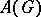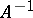# Open-mapping theorem

(diff) ← Older revision | Latest revision (diff) | Newer revision → (diff)
A continuous linear operatormapping a Banach spaceonto all of a Banach spaceis an open mapping, i.e.is open infor anywhich is open in. This was proved by S. Banach. Furthermore, a continuous linear operatorgiving a one-to-one transformation of a Banach spaceonto a Banach spaceis a homeomorphism, i.e.is also a continuous linear operator (Banach's homeomorphism theorem).
The conditions of the open-mapping theorem are satisfied, for example, by every non-zero continuous linear functional defined on a real (complex) Banach spacewith values in(in).
The open-mapping theorem can be generalized as follows: A continuous linear operator mapping a fully-complete (or-complete) topological vector spaceonto a barrelled spaceis an open mapping. The closed-graph theorem can be considered alongside with the open-mapping theorem.